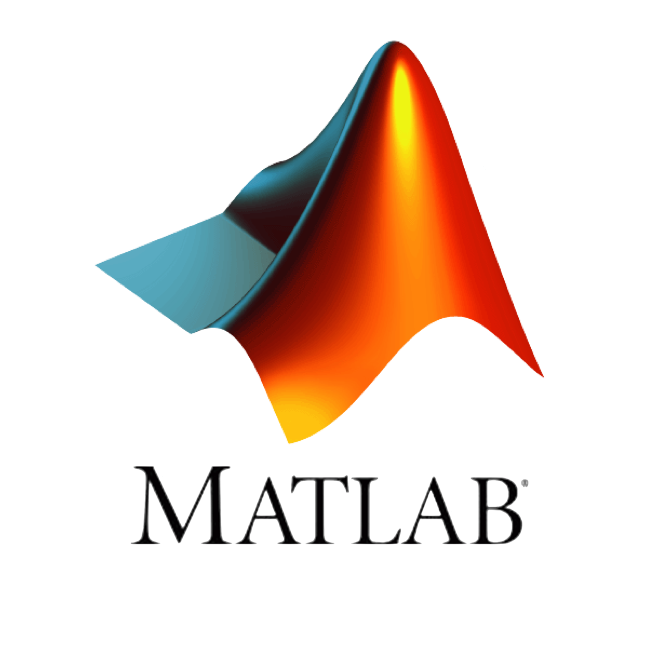# Introduction to MATLAB (Part 2)“Introduction to MATLAB (Part 2)” offers the opportunity to continue learning the basics of the MATLAB programming language. This course is designed as a continuation of the course “Introduction to MATLAB”. Participants will continue to learn the high-level programming language MATLAB – elementary
math functions, linear algebra functions, as well as graphing questions. Optimization issues will be considered: minimization, in the case of one, several variable functions, replacement by an orthogonal series of functions, and an approach to formulating the optimization problem. The basic issues of solving differential equations will also be covered, as well as creating applications with the app builder and calculating using parallel computing. Most of the features discussed come with built-in HPC (High-Performance Computing) computing capabilities.
Lecturer: Dr.Sc.Ing. Maris Tēraudus.

MATLAB is a high-level language and interactive environment for numerical computation, visualization, and programming.

Due to licensing policy, this course is offered to Riga Technical University staff and students only.

In order to receive a certificate of participation in the course, 75% of the lessons and homework must be completed.

## Purpose and tasks of the course

The aim of the course is to develop the skills of course participants in working with MATLAB software, so that the acquired knowledge can also be used in working with HPC.

1. To introduce participants to basic math and linear algebra functions in MATLAB.
2. Review optimization problems and solving differential equations in MATLAB.
3. See building apps from your own program using app building.

The training courses will take place in the form of interactive (collaborative) lectures, repeating what was told on your computer and asking questions.

## Topics

The following topics will be covered during the course:

• MATLAB functions for creating graphs;
• Optimization questions (minimization, minimization for a function of several variables, regression).
• Features with built-in HPC computing capabilities;
• Optimization questions (replacing a function with a series of orthogonal functions, defining an optimization problem, optimproblem, optimvar, solve);
• Review of linear algebra functions (with built-in HPC computing capabilities) MATLAB decompositions, determinants, and more.Elementary math functions (with built-in HPC computing capabilities) in MATLAB;
• Solving differential equations;
• App Building.